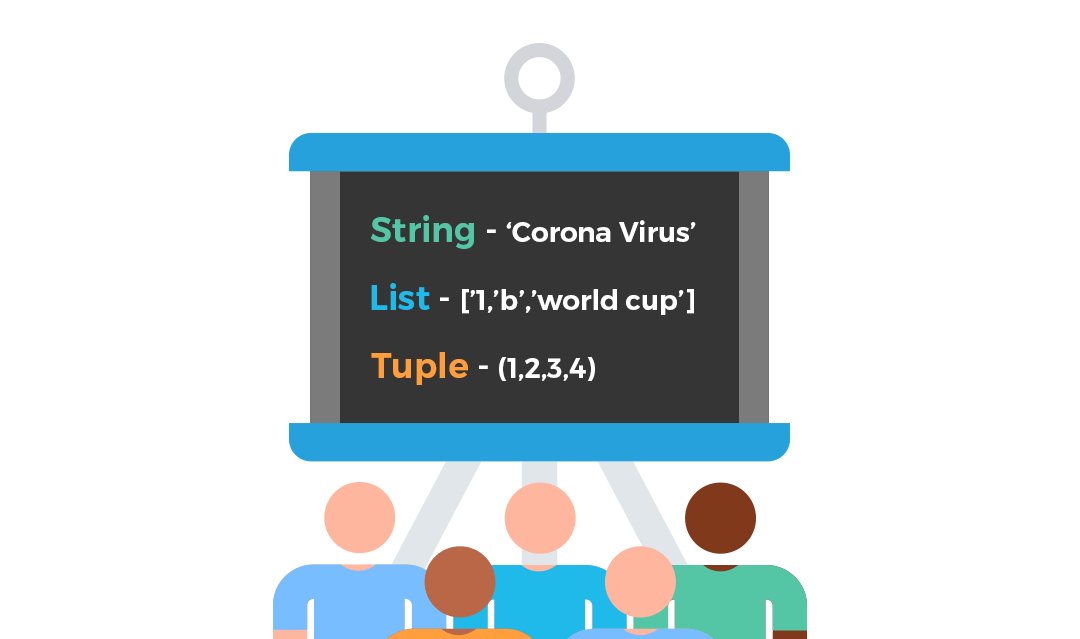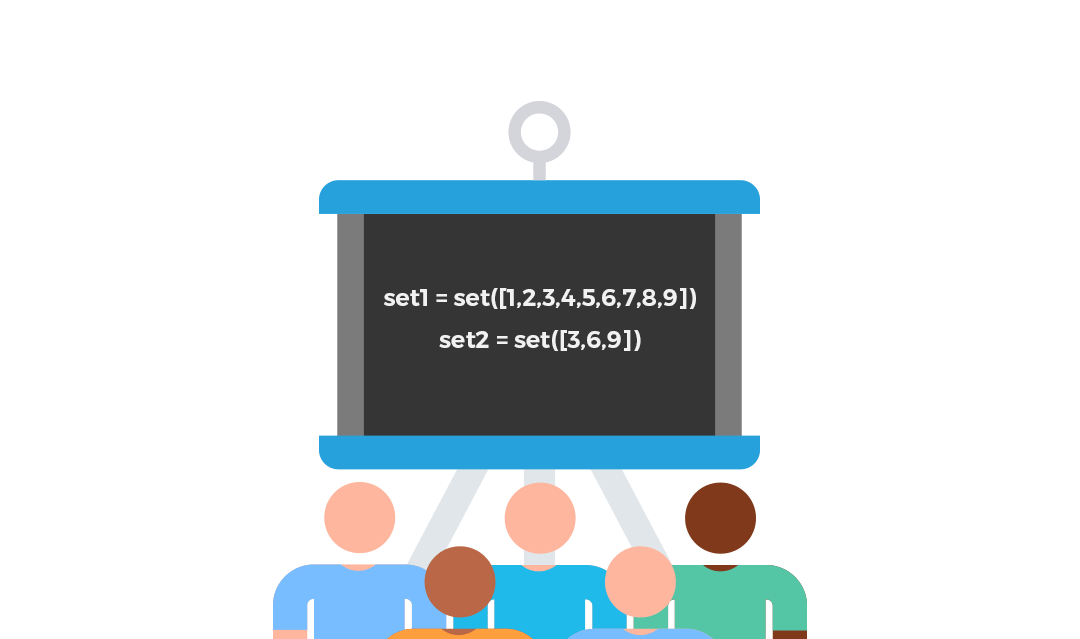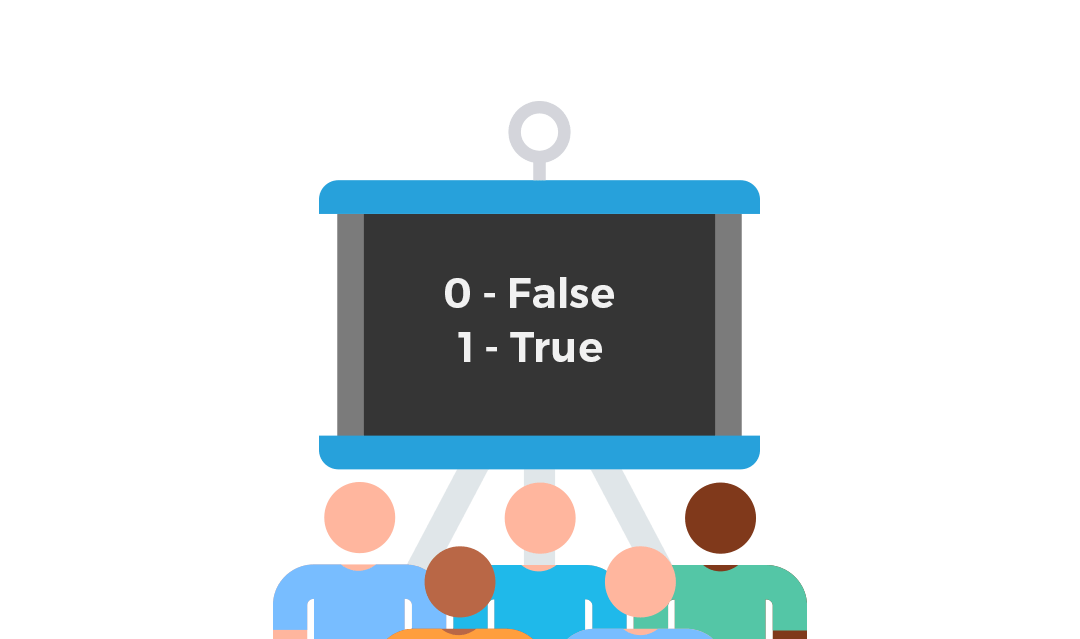# Top 5 Python Data Types that you should knowData types are the building blocks of every programming language. Any variable that stores a value contains some data which can be Boolean, string, number, etc. We talk in terms of classes and objects in Python. Data types are classes and the variables are objects. We can operate a variable based on what kind of data it has stored. In this article, we are going to explain all the data types available in Python and how we operate on them.

First of all, let us understand the rules to assign a variable.

• A variable can have a short name (like x and y) or a more descriptive name (age, car_name, total_volume)
• Name of a variable must start with a letter or an underscore character
• Do not start a variable name with a number
• A variable name can only contain alpha-numeric characters and underscores (A-z, 0-9, and _ )
• Variable names are case-sensitive (School_Name, school_name, and SCHOOL_NAME are three different variables)
• Variables cannot be named with words used in Python internally like for, in, not, list, tuple, dict, etc.

## Kind of Python Data Types –Python has 5 Standard data types which are listed below.

• Numeric
• Sequence Type
• Dictionary
• Sets
• Boolean

### Numeric –

When we store a numeric value in a variable, it automatically is classified as a numeric Python data type. There are 3 different numeric data types which are explained below.

1) Int (plain integers) – Any positive or negative whole number is called an integer and given int data type.

2) Float (floating point real values) – Real numbers with decimal values are called float.

3) Complex numbers – This type is used rarely in Python. A complex number is represented by X + iY where X and Y are floats and i is the square root of -1 (the result of which is an imaginary number)Please notePython 2 has two integer types – integer and a long integer. There is no ‘long integer’ in Python 3 anymore.

Now let us see how to work on this. We are defining three variables of different data types. In Python, we do not have to explicitly mention data type during the assignment of a variable. Applying the ‘Type’ function on a variable helps us identify the data type. We have used print command to get the result.

```standard = 10 # integer
car_loan_amount = 10000.90 # float

import cmath
complex_number = complex(10,20)
print(type(standard))
print(type(car_loan_amount))
print(type(complex_number))
print(complex_number.real)
print(complex_number.imag)
```

### Sequence Type –

There are 3 data types which comprise of a sequence of characters called string, or a sequence of mixed data types called a list or tuple.String –

A series of characters within a single, double or triple quotes is called a string. There is no character data type in Python like other languages. Let us see how to define a string variable along with its data type. In the below list of codes

```school_name = 'DPS' # str
print(type(school_name))

string_text = '''DPS is a very famous school''' # str
print(type(string_text))
```
Various operations can be performed on a string variable. For example,
1) Finding the length of the string using ‘len’ function
2) Accessing elements of a string variable
3) Reversing a string
4) Concatenating two strings
We are providing codes in serial order.
```#1 - length
len(string_text)
len(school_name)
#2 - Accessing the 6th element in the string
string_text

#2 - Accessing a sequence of elements
string_text[6:10]

#3 - Reversing the string
school_name[::-1]
string_text[::-1]

#4 - String concatenation
string_text + " " + school_name
```

List –

It is a compound data type. We can store various data types as elements in a list. To explain in other words, a list can consist of an integer, a string, as well as another list inside it. Every element in a list can be accessed with its index which starts with 0. A list is mutable. That is we can delete and modify its elements. Let us see how we can create a list.

```#creating a list
list1 = [1,2,'a','b','world cup']

#creating a nested list that is a list within a list
list2 = [1,2,'a','b','world cup', [1,2,'a','b','world cup']]
```
Various operations that can be performed on a list variable are as follows:

#### 1) Accessing elements of a list

As mentioned earlier, any element can be accessed using the index. We created a list named list1 above. Let us see how we can get to the various elements in it.
```#getting the element at the second index
print(list1)

#getting a sequence of elements from 1st to 3rd index can be made using [1:4] which follows [inclusion : exclusion] rule
print(list1[1:4])
```

#### 2) Appending an element or a list at the end

If we want to append a new element to list, we can do that by using the ‘append’ method and get the element added at the end of the list.
```#append function
list1.append('cricket')
print(list1)

#appending another list
list1.append(list2)
print(list1)
```

#### 3) Extending the list with an element or a list

Adding a new element at the end to extend the list is simple. It must be noted that the element or elements to be added must be placed as an iterable in the ‘extend’ method. The below commands will give you a clear idea.
```(list1.extend(list2))
print(list1)
```
Please note the difference between the outputs of append and extend from the screenshots.

#### 4) Finding the index of elements in a list

Many times we need to locate the index of a particular element in a list. We can identify that using the following command.
`list1.index('a')`

#### 5) Counting the occurrences of an element

If we want to count the occurrences of a particular element in the list, we can use the below command.
`list1.count('a')`

#### 6) Inserting an element at any index position

Insert takes in two arguments: insert(index, object) This method places the object at the index supplied.

```list1.insert(2,'inserted')
print(list1)
```

#### 7) Reversing a list

```list1.reverse()
print(list1)
```
Some other methods that we can apply are remove, pop, sort, etc.

### Tuple –

Python provides an immutable compound data type called Tuple. Similar to a list, a tuple can store elements of various data types but we can not edit it after creation. A few ways in which we can create a tuple are:

```#create an empty tuple
tuple()
t()
#create a tuple with 4 elements

t = (1,2,3,4)
print(t)
```

Some operations we can perform on a tuple are finding max, min, count, index, etc. to show a few methods. Let us see their commands.

```tup = (10,20,30,30,20,10,40,40,40,50,100)
print(tup.count(30))
print(tup.index(40))
print(min(tup))
print(max(tup))

```

### Dictionary –Another compound data type in Python is a dictionary. In this, we can save elements in a combination of key and value pairs and we can use this as a map. The syntax of a dictionary is { key1: value1, key2: value2, …. } . The command to create a dictionary is provided.

` dictionary1 = { 'name':'ivy' , 'class':'python', 'month':'march', 'year':2020} `
Some of the operations that we can perform on a dictionary are:

#### 1) Finding all the keys

We use method keys() on the dictionary variable.
` dictionary1.keys() `

#### 2) Finding all the values

We use method values().

` dictionary1.values() `

#### 3) Updating the value of a key

The Update method will add a new Key value pair if the key is not found. Else, it updates the value of the existing key.

```dictionary1.update({'batch':100})
print(dictionary1)
```

### Sets –Sets are a collection of unique similar objects. It is iterable and mutable. Sets can help us identify if an element exists or not in a highly optimized manner which is not the same in a list. We need to explicitly mention the term set while creating one. The values assigned to a set must be passed in a list or a tuple or any other data type.
```set1 = set([1,2,3,4])
type(set1)
```
A few methods we can apply to a set are
2) Removing an element
3) Identifying whether a set is a subset or a superset of another set
4) Union, Intersection, and difference between 2 sets
```#1 - adding
set1

#2 - removing
set1.remove(5)

#3 - subset/superset gives a true or false output
set1 = set([1,2,3,4,5,6,7,8,9])
set2 = set([3,6,9])
set1.issubset(set2)
set1.issuperset(set2)

#4 set operations
set1.union(set2)
set1.intersection(set2)
set1.difference(set2)
```
Please note that the slicing of a set does not exist as it does not support indexing.

### Boolean –It is another data type that contains one of the two in-built values True or False. We saw one example above.

## Conclusion –

All the various data types which one can require while working in Python have been explained. Please do let us know if there is any doubt regarding any command or data type. Next, we are going to discuss Loops in Python. Stay Tuned.!!

To know more about learning Python from our industry expert trainers, you can visit us at Ivy Professional School.